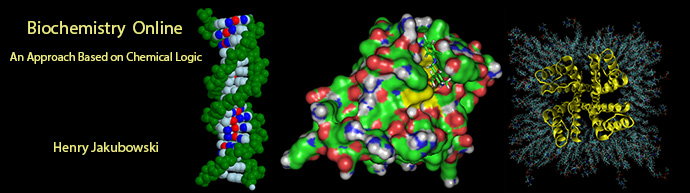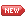# Biochemistry Online: An Approach Based on Chemical Logic# CHAPTER 9 - SIGNAL TRANSDUCTION

## F:  Signaling Math: Graphical Analyses of Inputs and Outputs

Wimot Osei-Bonsu and Henry Jakubowski11/16/17 New and slowly evolving .....

 Learning Goals/Objectives for Chapter 9F:After class and this reading, students will be able to describe the steady state in context of an isolated single enzyme-catalyzed reaction and for the enzyme when part of a complex metabolic pathway in vivo; state and describe what is required to perform a metabolic control analysis on a biological pathway; write ordinary differential equations to describe the change in concentration of analytes in a given set of coupled reactions; write mass balance expressions for Eo and use it to calculate the fraction of each enzyme form assuming rapid equilibrium; draw "wire" diagrams showing activation and inhibition for coupled reactions; define the flux control coefficient verbally and in the form of a mathematic equation, and explain how to obtain it graphically; use flux control coefficients to explain how control of flux in a pathway is distributed through all enzyme-catalyzed reactions in that pathway; analyze the result of a flux control analysis of a pathway like glycolysis; describe why small changes in the enzymes that have the greatest ΔG values in glycolysis have minor effects in the metabolic flux in glycolysis; define the concentration control coefficient verbally and in the form of a mathematic equation analyze the result of a concentration control analysis of a pathway like glycolysis; define the elasticity coefficient verbally and in the form  form of a mathematic equation, and explain how to obtain it graphically state whether the different metabolic control coefficients are global properties of the system or local properties of a given enzyme.Navigation